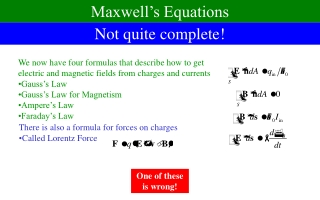DownloadDownload PresentationNot quite complete!

# Not quite complete!

Télécharger la présentation## Not quite complete!

- - - - - - - - - - - - - - - - - - - - - - - - - - - E N D - - - - - - - - - - - - - - - - - - - - - - - - - - -
##### Presentation Transcript

1. Maxwell’s Equations Not quite complete! • We now have four formulas that describe how to get electric and magnetic fields from charges and currents • Gauss’s Law • Gauss’s Law for Magnetism • Ampere’s Law • Faraday’s Law • There is also a formula for forces on charges • Called Lorentz Force One of these is wrong!

2. Ampere’s Law is Wrong! • Maxwell realized Ampere’s Law is not self-consistent • This isn’t an experimental argument, but a theoretical one • Consider a parallel plate capacitor getting charged by a wire • Consider an Ampere surface between the plates • Consider an Ampere surface in front of plates • But they must give the same answer! • There must be something else that creates B-fields • Note that for the first surface, there is also an electric field accumulating in capacitor • Maybe electric fields? • Take the time derivative of this formula • Speculate : This replaces I for first surface I I

3. Ampere’s Law (New Recipe) I2 I1 E1 E2 • Is this self-consistent? • Consider two surfaces with the same boundary • Gauss’s Law for electric fields: B • This makes sense!

4. Maxwell’s Equations • This is not the form in which Maxwell’s Equations are usually written • It involves complicated integrals • It involves long-range effects • Our first goal – rewrite them as local equations • Make the volumes very small • Make the loops very small • Large volumes and loops can be made from small ones • If it works on the small scale, it will work on the large Skip slides

5. Gauss’s Law for Small Volumes (2) a a a z y x • Consider a cube of side a • One corner at point (x,y,z) • a will be assumed to be very small • Gauss’s Law says: • Let’s get flux on front and back face: • Now include the other four faces:

6. Gauss’s Law for Small Volumes a a a • Divide both sides by a3, the volume • q/V is called charge density  • A similar computation works for Gauss’s Law for magnetic fields: • A more mathematically sophisticated notation allows you to write these more succinctly:

7. Ampere’s Law for Small Loops • Consider a square loop a • One corner at point (x,y,z) • a will be assumed to be very small • Ampere’s Law says: • Let’s get integral on top and bottom z a y x a • Add the left and right sides • Calculate the electric flux • Put it together

8. Ampere’s Law for Small Loops (2) z a y x • Divide by a2 • Current density J is I/A • Only in x-direction counts • Redo it for loops oriented in the other two directions • Similar formulas can be found for Faraday’s Law a

9. Maxwell’s Equations: Differential Form • In more sophisticated notation: